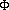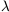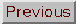### SCALAR FIELD

Adapted from P. Coles, 1999, The Routledge Critical Dictionary of the New Cosmology, Routledge Inc., New York. Reprinted with the author's permission. To order this book click here: http://www.routledge-ny.com/books.cfm?isbn=0415923549

In the standard Friedmann models on which the Big Bang theory is based, the material components of the Universe are generally described as if they were perfect classical fluids with a well-defined densityand pressure p. Models of such perfect fluids can describe most of the thermal history of the Universe quite adequately, but for the very early Universe they are expected to break down. At very high temperatures it is necessary to describe matter using quantum field theory rather than fluid mechanics, and this requires some alterations to be made to the relevant cosmological models.

One idea which emerges from these considerations, and which is now ubiquitous in modern cosmology, is the idea that the dynamical behaviour of the early Universe might be dominated by a variety of quantum field called a scalar field. A scalar field is characterised by some numerical value, which we shall call. It can be a function of spatial position, but for the purposes of illustration we take it to be a constant. (A vector field would be characterised by a set of numbers for each spatial position, like the different components of spin, for example.) We can discuss many aspects of this kind of entity without having to use detailed quantum theory by introducing the concept of a Lagrangian action to describe its interactions. The Lagrangian for a scalar field can be written in the form

L() = 1/2 (d/ dt)2 - V()

The first of these terms is usually called the kinetic term (it looks like the square of a velocity), while the second is the potential term (V is a function that describes the interactions of the field). The Lagrangian action is used to derive the equations that show howvaries with time, but we do not need them for this discussion. The appropriate energy-momentum tensor to describe such a field in the framework of general relativity can be written in the form

Tij = pgij + (p +)Ui Uj

where gij is the metric, and Ui and Uj are components of the 4-velocity. To simplify this equation, and the following ones, we have introduced a convention from particle physics in which h / 2= c = 1. The energy densityand pressure p in this expression are effective quantities, given by= 1/2 (d/ dt)2 + V()

and

p = 1/2 (d/ dt)2 - V()

If the kinetic term is negligible with respect to the potential term, the effective equation of state for the field becomes p = -. This is what happens during the phase transitions that are thought the drive the inflationary Universe model. Under these conditions the field behaves in exactly the same way as an effective cosmological constant with= 8G/ c2

where we have replaced the required factor of c2 in this expression.

Despite the fact that the scalar field can behave as a fluid in certain situations, it is important to realise that it is not like a fluid in general. Ifis oscillating, for example, there is no definite relationship between the effective pressure and the effective energy density.

FURTHER READING: Kolb, F.W. and Turner, M.S., The Early Universe (Addison-Wesley, Redwood City, CA, 1990).